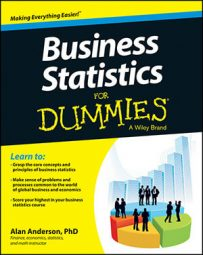When you use a small sample to test a hypothesis about a population mean, you take the resulting critical value or values from the Student's t-distribution. For a two-tailed test, the critical value is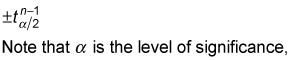and n represents the sample size.

The Student's t-distribution
Degrees of Freedom t0.10 t0.05 t0.025 t0.01 t0.005
6 1.440 1.943 2.447 3.143 3.707
7 1.415 1.895 2.365 2.998 3.499
8 1.397 1.860 2.306 2.896 3.355
9 1.383 1.833 2.262 2.821 3.250
10 1.372 1.812 2.228 2.764 3.169
11 1.363 1.796 2.201 2.718 3.106
12 1.356 1.782 2.179 2.681 3.055
13 1.350 1.771 2.160 2.650 3.012
14 1.345 1.761 2.145 2.624 2.977
15 1.341 1.753 2.131 2.602 2.947

The number of degrees of freedom used with the t-distribution depends on the particular application. For testing hypotheses about the population mean, the appropriate number of degrees of freedom is one less than the sample size (that is, n – 1).

The critical value or values are used to locate the areas under the curve of a distribution that are too extreme to be consistent with the null hypothesis. For a two-tailed test, the value of the level of significance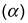is split in half; the area in the right tail equals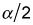and the area in the left tail equals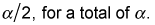As an example of a two-tailed test, suppose the level of significance is 0.05 and the sample size is 10; then you get a positive and a negative critical value: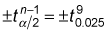You can get the value of the positive critical value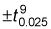directly from the Student's t-distribution table.

In this case, you find the positive critical value t90.025 at the intersection of the row representing 9 degrees in the Degrees of Freedom column and the t0.025 column. The positive critical value is 2.262; therefore, the negative critical value is –2.262. You represent these two values like so: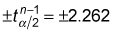You represent them graphically as shown here.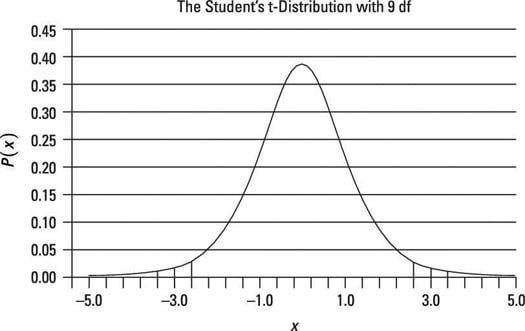Critical value taken from the t-distribution: two-tailed test.

The shaded region in the two tails represents the rejection region; if the test statistic falls in either tail, the null hypothesis will be rejected.